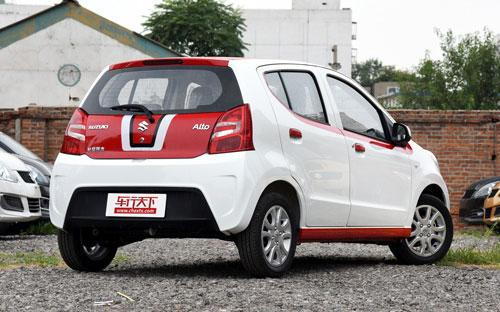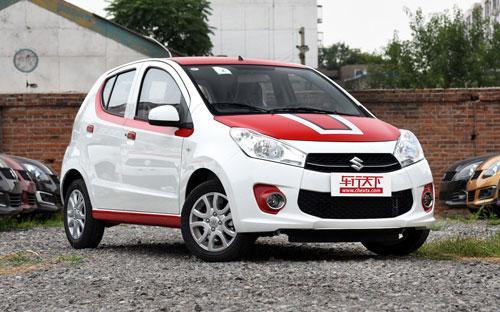### 长安铃木 奥拓5 种颜色可选2015款最低售价：5.29 万元起

3570(mm)1600(mm)1470(mm)##### 配置亮点：
• 胎压监测装置

• ISOFIX儿童座椅接口

• 车身稳定控制(ESC/ESP/DSC等)

• 电动天窗

• 定速巡航

• 后倒车雷达

• 真皮座椅

• GPS导航系统

• 氙气大灯

• 后视镜加热

• 提交
2015款 1.0L 手动限定型 (99张)

长安铃木 奥拓 绕车实拍• 长安铃木 奥拓 在售车型

排量 车型 厂商指导价 本地最低报价 购车工具
1.0L
5.29万
5.29万

6.19万
6.19万

长安铃木 奥拓 经销商

查看更多 >>

### 长安铃木 奥拓 动力加速

奥拓 0-100公里加速时间分布在 0.0-秒 属于 超跑级

动力级别 加速时间 车型

长安铃木 奥拓 视频

长安铃木 奥拓 新闻资讯

# 售5.29万起 铃木雨燕/奥拓限定款上市

新闻 超过8266次关注

日前，我们从长安铃木官方获悉，雨燕和奥拓车型推出了新的限定款车型，新车的最大特点是采用定制双色的配色，雨燕限定款的价格区间为7.28-8.28万元。奥拓限定款的价...

# 豪华型值得购买 2013款奥拓购车手册

车系文章 超过3142次关注

看到这篇文章的时候，相信许多人心里都有这么一个疑问，2013款奥拓不是上市许久了么，怎么现在还出购车手册？理由很简单，目前它在微型车里的关注度位列第一。从197...

# 2013款铃木奥拓到店实拍 外观变化最大

车系文章 超过2782次关注

长安铃木在成都车展宣布2013款新奥拓正式上市。此次共推出了豪华型和炫酷型2个系列6款车型。而作为中期改款车型，新奥拓在外观方面的变化最大，而我们也第一时间到...

# 试城市精品小车全新奥拓 感受1.0L+4AT

车系文章 超过2772次关注

经历过上世纪90年代的无论年幼老少，相信不会有人忘记这样一台小车，它对于中国家轿的意义不亚于桑塔纳与捷达，它曾经叱咤国内微型车市场超过10年，它就是奥拓！不...

# 奥拓电动版国内谍照曝光 或于年底发布

车系文章 超过2300次关注

节能减排已经成为汽车市场的趋势，因此电动车已经不再是部分品牌的专属。近日，国内媒体曝光了一组长安铃木奥拓电动版国内路试谍照，新车有望于今年年底发布。

# 售5.24-6.14万 奥拓20周年限量版上市

车系文章 超过2232次关注

日前，长安铃木正式推出奥拓20周年限量版车型，此次上市的新车共有两款车型，价格区间为5.24-6.14万元。

# 长安铃木召回新奥拓/雨燕等部分车型

碰撞召回 超过2476次关注

日前，重庆长安铃木汽车有限公司按照《缺陷汽车产品召回管理条例》的要求，向国家质检总局备案了召回计划，决定在2013年5月31日起召回部分新奥拓、雨燕、羚羊、天语...

猜你喜欢

﻿
• 快速找车
• 选择品牌
• 选择品牌
• A  奥迪
• A  阿斯顿·马丁
• A  阿尔法·罗密欧
• B  宝沃
• B  布加迪
• B  巴博斯
• B  保时捷
• B  宾利
• B  奔驰
• B  宝马
• B  本田
• B  别克
• B  标致
• B  比亚迪
• B  宝骏
• B  北汽制造
• B  北汽新能源
• B  北汽幻速
• B  北汽威旺
• B  北京汽车
• B  奔腾
• B  北汽绅宝
• C  长安
• C  长安商用
• C  长城
• C  昌河
• D  大众
• D  道奇
• D  DS
• D  东南
• D  东风风神
• D  东风风行
• D  东风小康
• D  东风风度
• D  东风
• F  福特
• F  丰田
• F  菲亚特
• F  法拉利
• F  福田
• F  福迪
• F  福汽启腾
• G  观致
• G  广汽传祺
• G  广汽吉奥
• G  GMC
• H  红旗
• H  汉腾汽车
• H  哈弗
• H  哈飞
• H  海格
• H  海马
• H  华颂
• H  黄海
• H  华泰
• H  恒天
• J  吉利汽车
• J  捷豹
• J  Jeep
• J  江淮
• J  江铃
• J  金杯
• J  九龙
• J  金旅
• K  凯翼
• K  凯迪拉克
• K  克莱斯勒
• K  科尼塞克
• K  卡威
• K  开瑞
• L  路虎
• L  林肯
• L  劳斯莱斯
• L  兰博基尼
• L  雷克萨斯
• L  铃木
• L  雷诺
• L  理念
• L  力帆
• L  莲花汽车
• L  猎豹
• L  路特斯
• L  陆风
• M  马自达
• M  MG
• M  MINI
• M  玛莎拉蒂
• M  摩根
• M  迈凯轮
• N  纳智捷
• O  欧宝
• O  讴歌
• O  欧朗
• Q  奇瑞
• Q  起亚
• Q  启辰
• R  日产
• R  荣威
• R  瑞麒
• S  三菱
• S  斯威汽车
• S  萨博
• S  smart
• S  斯柯达
• S  斯巴鲁
• S  思铭
• S  双龙
• S  上汽大通
• S  双环
• T  特斯拉
• T  腾势
• W  沃尔沃
• W  五菱汽车
• W  五十铃
• W  威兹曼
• W  威麟
• X  现代
• X  雪佛兰
• X  雪铁龙
• X  西雅特
• Y  一汽
• Y  英菲尼迪
• Y  英致
• Y  依维柯
• Y  野马汽车
• Y  永源
• Z  众泰
• Z  中华
• Z  中兴
• Z  知豆
• 选择车系
• 选择车系
• 车型对比
• 选择品牌
• 选择品牌
• A  奥迪
• A  阿斯顿·马丁
• A  阿尔法·罗密欧
• B  宝沃
• B  布加迪
• B  巴博斯
• B  保时捷
• B  宾利
• B  奔驰
• B  宝马
• B  本田
• B  别克
• B  标致
• B  比亚迪
• B  宝骏
• B  北汽制造
• B  北汽新能源
• B  北汽幻速
• B  北汽威旺
• B  北京汽车
• B  奔腾
• B  北汽绅宝
• C  长安
• C  长安商用
• C  长城
• C  昌河
• D  大众
• D  道奇
• D  DS
• D  东南
• D  东风风神
• D  东风风行
• D  东风小康
• D  东风风度
• D  东风
• F  福特
• F  丰田
• F  菲亚特
• F  法拉利
• F  福田
• F  福迪
• F  福汽启腾
• G  观致
• G  广汽传祺
• G  广汽吉奥
• G  GMC
• H  红旗
• H  汉腾汽车
• H  哈弗
• H  哈飞
• H  海格
• H  海马
• H  华颂
• H  黄海
• H  华泰
• H  恒天
• J  吉利汽车
• J  捷豹
• J  Jeep
• J  江淮
• J  江铃
• J  金杯
• J  九龙
• J  金旅
• K  凯翼
• K  凯迪拉克
• K  克莱斯勒
• K  科尼塞克
• K  卡威
• K  开瑞
• L  路虎
• L  林肯
• L  劳斯莱斯
• L  兰博基尼
• L  雷克萨斯
• L  铃木
• L  雷诺
• L  理念
• L  力帆
• L  莲花汽车
• L  猎豹
• L  路特斯
• L  陆风
• M  马自达
• M  MG
• M  MINI
• M  玛莎拉蒂
• M  摩根
• M  迈凯轮
• N  纳智捷
• O  欧宝
• O  讴歌
• O  欧朗
• Q  奇瑞
• Q  起亚
• Q  启辰
• R  日产
• R  荣威
• R  瑞麒
• S  三菱
• S  斯威汽车
• S  萨博
• S  smart
• S  斯柯达
• S  斯巴鲁
• S  思铭
• S  双龙
• S  上汽大通
• S  双环
• T  特斯拉
• T  腾势
• W  沃尔沃
• W  五菱汽车
• W  五十铃
• W  威兹曼
• W  威麟
• X  现代
• X  雪佛兰
• X  雪铁龙
• X  西雅特
• Y  一汽
• Y  英菲尼迪
• Y  英致
• Y  依维柯
• Y  野马汽车
• Y  永源
• Z  众泰
• Z  中华
• Z  中兴
• Z  知豆
• 选择车系
• 选择车系
• 选择车型
• 选择车型
• 意见反馈